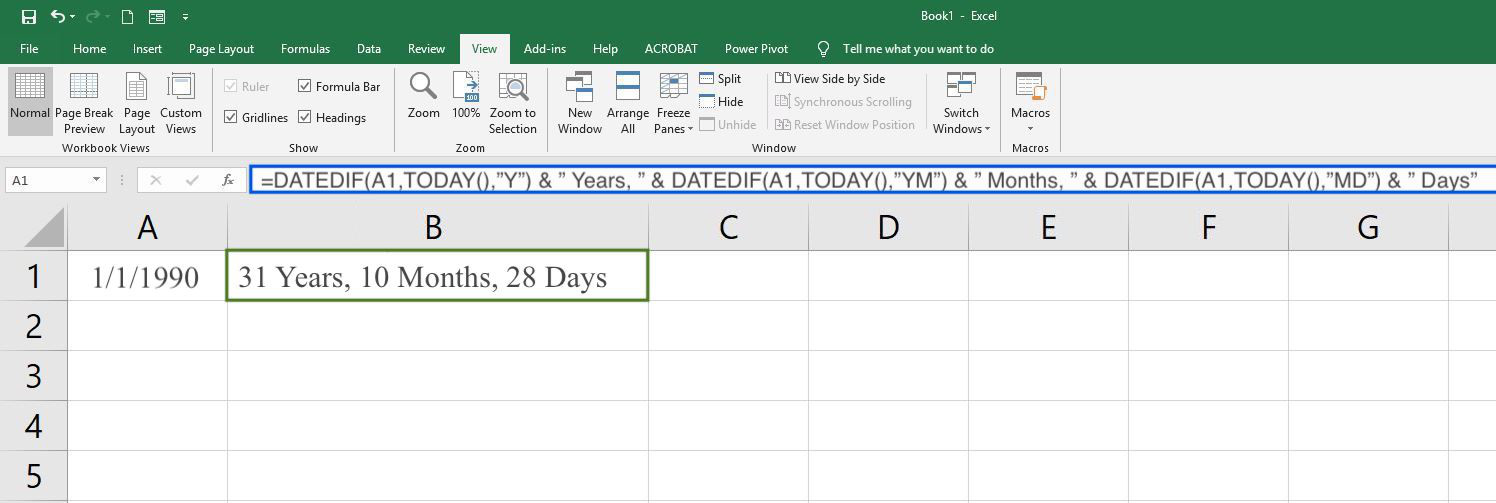# Age Calculator – Calculate Your Exact Age

Calculate how old you are using the age calculator below. Get your age in years, months, days, or seconds.

Birthdate:
Age at this Date:

 Age in Months: Age in Days: Age in Hours: Age in Minutes: Age in Seconds: Next Birthday:
Learn how we calculated this below

## How Old Are You?

Ever want to know your age in days? Maybe not, but our age calculator can find the age in years, months, days, hours, minutes, and seconds too!

Enter the date of birth and the date to compare it with, and the calculator will find the age on that date, along with the days until the next birthday.

## How to Calculate Your Age

You can find out how old you are without using an age calculator by subtracting the current year from your birth year. Then, if how haven’t already had a birthday yet this year, subtract one from your age.

age in years = current year – birth year

### How Many Days Old You Are?

Are you wondering how many days old am I? The most accurate way to find out how old you are in days is to use an age calculator since it accounts for things like leap years.

But, you can also calculate how old you are in days by multiplying one plus your age by 365.25. Then, subtract the number of days until your next birthday.

age in days = ((age in years + 1) × 365.25) – days until birthday

This formula uses 365.25 days per year to account for leap years, where every four years there is an extra day.

For example, if you are 23 years old and your birthday is in 45 days, you can find out how many days old you are using the following formula:

age in days = ((23 + 1) × 365.25) – 45
age in days = (24 × 365.25) – 45
age in days = 8766 – 45
age in days = 8721

So, you would be 8721 days old using this formula.

## How to Calculate Age using Excel

The Excel program also has a built-in age calculator that you can use to find out how old you are. To use it, enter your birthday using the format MM/DD/YYYY in cell A1.

Then, use the function editor to enter the following function in cell B1:

=DATEDIF(A1,TODAY(),”Y”) & ” Years, ” & DATEDIF(A1,TODAY(),”YM”) & ” Months, ” & DATEDIF(A1,TODAY(),”MD”) & ” Days”

This function will display your age in years, months, and days.Next Up – Find out how old your pet is using our cat and dog age calculators.